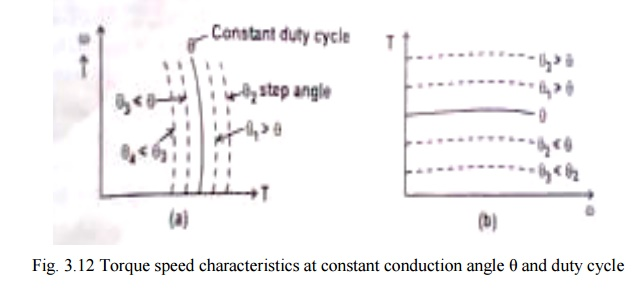Home | | Special Electrical Machines | Torque-Speed Characteristics of SRM(Switched Reluctance Motor)

# Torque-Speed Characteristics of SRM(Switched Reluctance Motor)

Torque Speed Capability Curve

TORQUE-SPEED CHARACTERISTICS

Torque developed (i.e.) average torque developed but SRM depends upon the current wave form of SRM phase winding. Current waveform depends upon the conduction period and chopping details. It also depends upon the speed.

Consider a case that conduction angle ϴ is constant and the chopper duty cycle is 1.(i.e.) it conducts continuously. For low speed operating condition, the current is assumed to be almost flat shaped. Therefore the developed torque is constant. For high speed operating condition, the current wave form gets changed and the average torque developed gets reduced.

Fig. 3.12(a) represents the speed torque characteristics of SRM for constant ϴ and duty cycle. It is constant at low speeds and slightly droops as speed increases. For various other constant value of ϴ , the family of curves for the same duty cycle is shown in fig.3.12.Torque speed characteristics for fixed ϴ and for various duty cycles are shown in fig. 3.12. ϴ and duty cycle are varied by suitably operating the semiconductor devices.

1. Torque Speed Capability Curve

Maximum torque developed in a motor and the maximum power that can be transferred are usually restricted by the mechanical subsystem design parameters.

For given conduction angle the torque can be varied by varying the duty cycle of the chopper. However the maximum torque developed is restricted to definite value based on mechanical consideration.AB in the fig.3.13 represents constant maximum torque region of operation.

At very low speeds, the torque / speed capability curve may deviate from the clock torque characteristics. If the chopping frequency is limited or if the bandwidth of the current regulator is limited, it is difficult to limit the current without the help of self emf of the motor and the current reference may have to be reduced.

If very low windage and core loss permit the chopper losses to be increased, so that with higher current a higher torque is obtained. Under intermittent condition of course very much higher torque can be obtained in any part of the speed range up to Ѡ b.

The motor current limits the torque below base speed. The ‗corner point‘ or base speed ‗Ѡ b‘ is the highest speed at which maximum current can be supplied at rated voltage with fixed firing angles. If these angles are still kept fixed, the maximum torque at rated voltage decreases with speed squared. But if the conduction angle is increased,(i.e.)ϴ on is decreased, there is a considerable speed range over which maximum current can be still be forced into the motor. This maintains the torque at a higher level to maintain constant power characteristic. But the core losses and windage losses increases with the speed. Thus the curve BC represents the maximum permissible torque at each speed without exceeding the maximum permissible power transferred. This region is obtained by varying ϴ D to its maximum value ϴ D max. ϴ D is dwell angle of the main switching devices in each phase. Point C corresponds to maximum permissible power; maximum permissible conduction angle

ϴ D max and duty cycle of the chopper is unity.

Curve CD represents TѠ 2 constant. The conduction angle is kept maximum and duty cycle is maximum by maintaining TѠ 2 constant. D corresponds to maximum Ѡ permissible.

The region between the curve ABCD and X axis is the ―permissible region of operation of SRM‖.

Study Material, Lecturing Notes, Assignment, Reference, Wiki description explanation, brief detail
Special Electrical Machines : Switched Reluctance Motor : Torque-Speed Characteristics of SRM(Switched Reluctance Motor) |